# Topic 7 Linear RegressionIntroduction

• Regression analysis is one of the most widely used tool in quantitative research which is used to analyse the relationship between variables.

• One or more variables are considered to be explanatory variables, and the other is considered to be the dependent variable.

• In general linear regression is used to predict a continuous dependent variable (regressand) from a number of independent variables (regressors) assuming that the relationship between the dependent and independent variables is linear.

• If we have a dependent (or response) variable Y which is related to a predictor variables $$X_{i}$$. The simple regression model is given by

$\begin{equation} Y=\alpha+\beta X_{i}+\epsilon_{i} \tag{7.1} \end{equation}$

• R has the function $$\mathtt{lm}$$ (linear model) for linear regression.

• The main arguments to the function $$\mathtt{lm}$$ are a formula and the data. $$\mathtt{lm}$$ takes the defining model input as a formula

• A formula object is also used in other statistical function like $$\mathtt{glm,\,nls,\,rq}$$ etc, which is from a formula class.

## 7.1 Investment $$\beta$$ using R (Single Index Model)

The ‘market model’ regression can be represented as the following regression.

$\begin{equation} R_{i}=\alpha+\beta_{i}R_{M}+\epsilon \tag{7.2} \end{equation}$

## 7.2 Data preprocessing

• Convert data to returns

• Generate some descriptive statistics

• Some plots

• Data

# Run the following to download and save the data, this should be
# done once and when updating the time period
library(quantmod)
library(pander)
library(xts)
library(TTR)
BHP = getSymbols("BHP.AX", from = "2019-01-01", to = "2021-07-31", auto.assign = FALSE)
ASX = getSymbols("^AXJO", from = "2019-01-01", to = "2021-07-31", auto.assign = FALSE)
# save both in rds (to be used in the TA chapter)
saveRDS(BHP, file = "data/bhp_prices.rds")
saveRDS(ASX, file = "data/asx200.rds")
• Convert to returns
library(quantmod)
library(pander)
library(xts)
library(TTR)
# load data from the saved files (not required if we execute the
# chunk above)
# using close prices
bhp2 = BHP$BHP.AX.Close asx2 = ASX$AXJO.Close
# covert to returns

bhp_ret = dailyReturn(bhp2, type = "log")
asx_ret = dailyReturn(asx2, type = "log")

# merge the two with 'inner' join to get the same dates
data_lm1 = merge.xts(bhp_ret, asx_ret, join = "inner")
# convert to data frame
data_lm2 = data.frame(index(data_lm1), data_lm1$daily.returns, data_lm1$daily.returns.1)
# change column names
colnames(data_lm2) = c("Date", "bhp", "asx")
head(data_lm2)  #there are row names which can be removed if required
                 Date          bhp          asx
2019-01-02 2019-01-02  0.000000000  0.000000000
2019-01-03 2019-01-03  0.000000000  0.013510839
2019-01-04 2019-01-04 -0.008947241 -0.002488271
2019-01-07 2019-01-07  0.029808847  0.011289609
2019-01-08 2019-01-08  0.001162482  0.006873792
2019-01-09 2019-01-09 -0.003782952  0.009721207
library(pastecs)
desc_stat1 = stat.desc(data_lm2[, 2:3], norm = TRUE)
pander(desc_stat1, caption = "Descriptive Statistics", split.table = Inf)
Descriptive Statistics
bhp asx
nbr.val 653 653
nbr.null 9 1
nbr.na 0 0
min -0.1557 -0.102
max 0.1128 0.06766
range 0.2685 0.1697
sum 0.4948 0.2853
median 0 0.001163
mean 0.0007577 0.0004369
SE.mean 0.0007444 0.0005075
CI.mean.0.95 0.001462 0.0009966
var 0.0003618 0.0001682
std.dev 0.01902 0.01297
coef.var 25.1 29.69
skewness -0.4883 -1.443
skew.2SE -2.553 -7.546
kurtosis 9.991 13.32
kurt.2SE 26.16 34.86
normtest.W 0.9198 0.8266
normtest.p 3.885e-18 5.497e-26

## 7.3 Visualisation

library(ggplot2)
library(tidyr)

p1 = ggplot(data_lm2, aes(asx, bhp))
p1 + geom_point(colour = "brown") + geom_smooth(method = "lm") + theme_minimal() +
labs(title = "Scatter plot of BHPvsASX and Linear Fit")p2 = ggplot(data_lm2, aes(Date))
p2 + geom_line(aes(y = bhp, color = "bhp"), size = 1, lty = 1) + geom_line(aes(y = asx,
color = "asx"), size = 1, lty = 2) + scale_color_discrete("Asset") +
theme_minimal() + labs("Line Chart of Returns")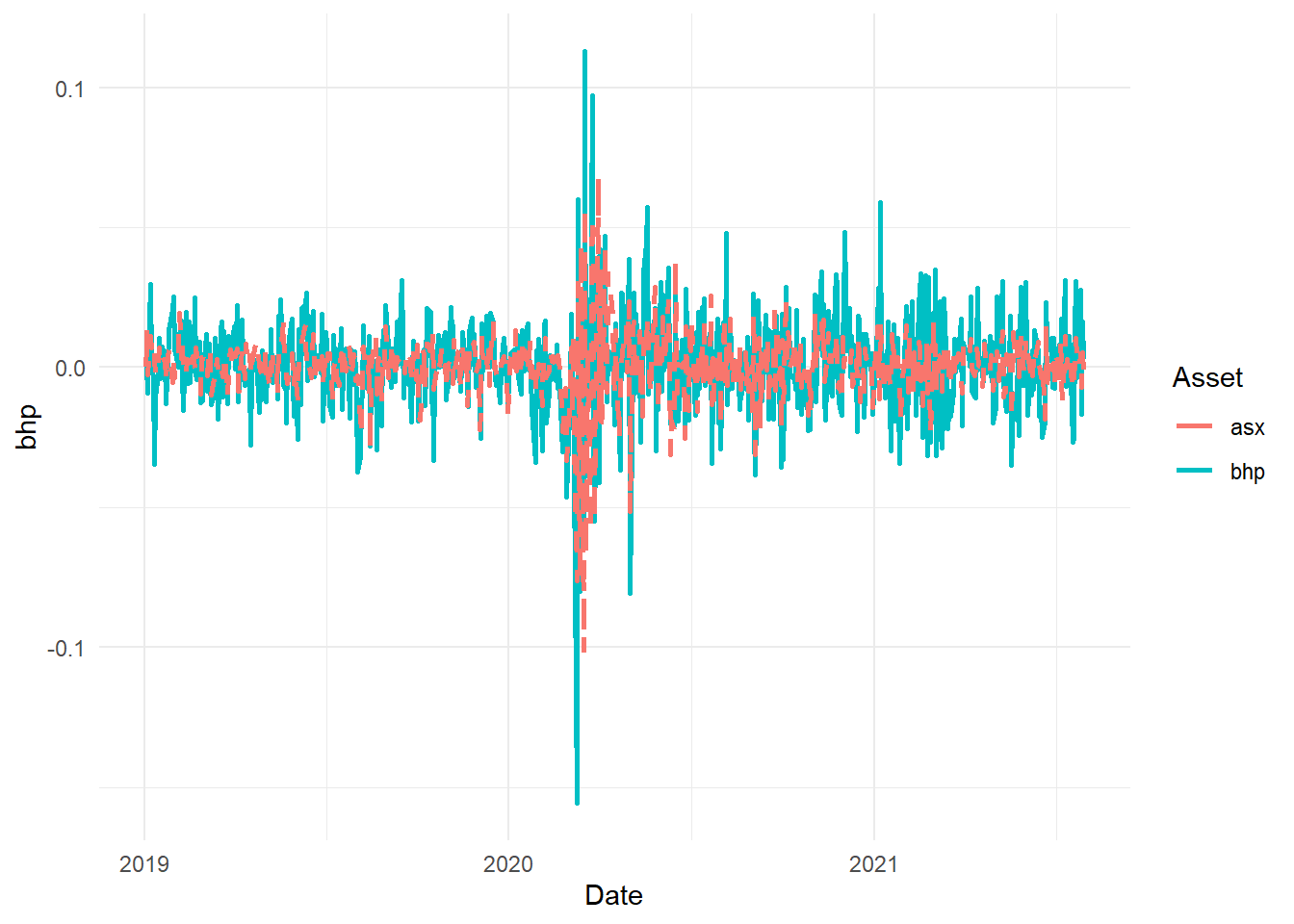## 7.4 Regression analysis using lm

• Use lm to model the SIM
lreg1 = lm(formula = bhp ~ asx, data = data_lm2)
summary(lreg1)  #to generate main results

Call:
lm(formula = bhp ~ asx, data = data_lm2)

Residuals:
Min        1Q    Median        3Q       Max
-0.077007 -0.008153 -0.000162  0.007500  0.060409

Coefficients:
Estimate Std. Error t value Pr(>|t|)
(Intercept) 0.0003046  0.0005270   0.578    0.564
asx         1.0372279  0.0406422  25.521   <2e-16 ***
---
Signif. codes:  0 '***' 0.001 '**' 0.01 '*' 0.05 '.' 0.1 ' ' 1

Residual standard error: 0.01346 on 651 degrees of freedom
Multiple R-squared:  0.5001,    Adjusted R-squared:  0.4994
F-statistic: 651.3 on 1 and 651 DF,  p-value: < 2.2e-16
pander(lreg1, add.significance.stars = T)  #to tabulate
Fitting linear model: bhp ~ asx
Estimate Std. Error t value Pr(>|t|)
(Intercept) 0.0003046 0.000527 0.5779 0.5635
asx 1.037 0.04064 25.52 4.215e-100 * * *
• Using stargazer to print the output
library(stargazer)
stargazer(lreg1, type = "html", title = "Regression Results")
 Dependent variable: bhp asx 1.037*** (0.041) Constant 0.0003 (0.001) Observations 653 R2 0.500 Adjusted R2 0.499 Residual Std. Error 0.013 (df = 651) F Statistic 651.320*** (df = 1; 651) Note: p<0.1; p<0.05; p<0.01
• Diagnostic Plots
par(mfrow = c(2, 2))
plot(lreg1)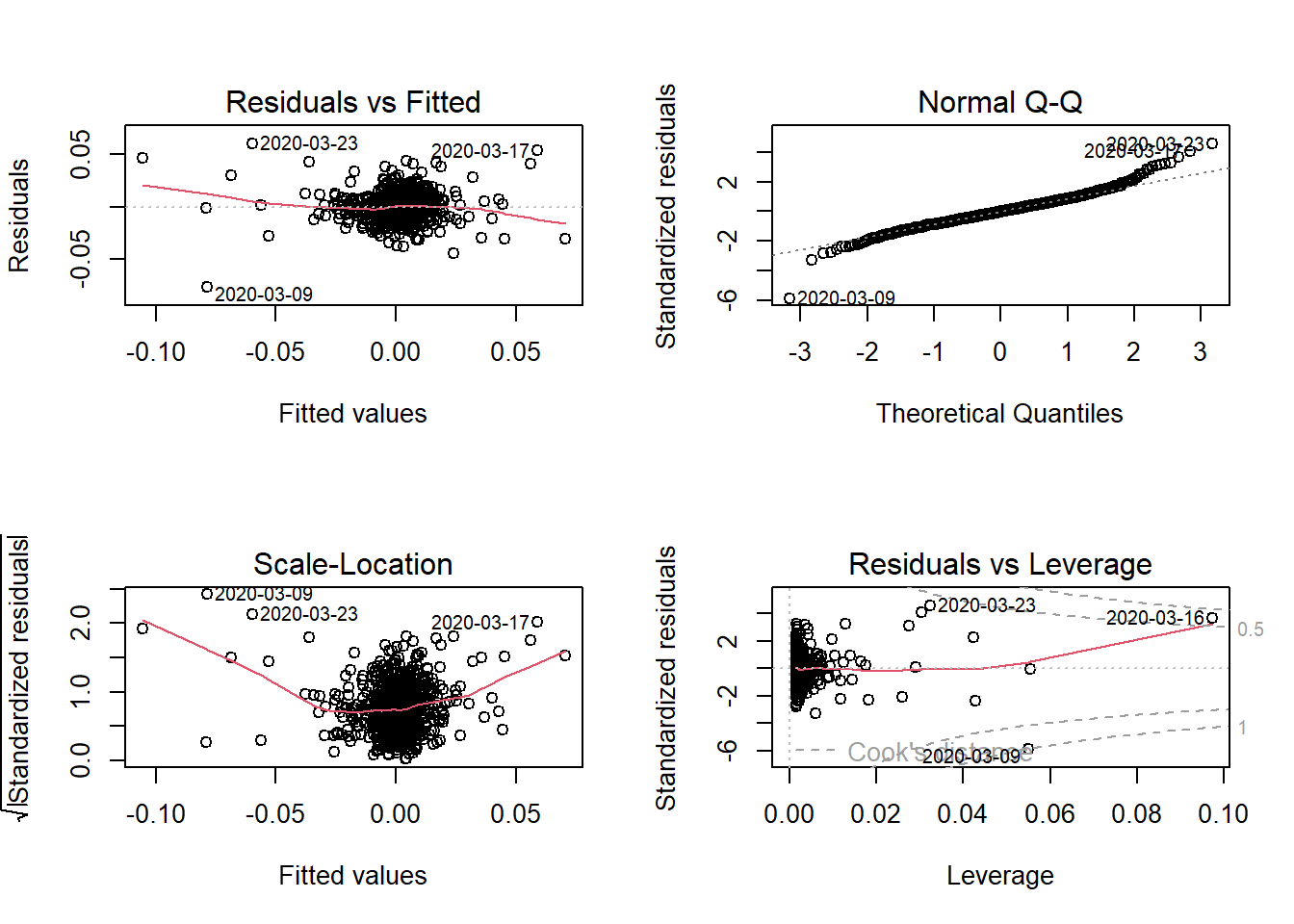# Topic 8 Multiple Regression

• Multiple regression extends simple linear regression with more than one (1) predictor variables
• We have one response variable and multiple independent predictor variable.

$\begin{equation} y_{i}=\alpha+\beta_{1}x_{1}+\beta_{2}x_{2}+\ldots+\varepsilon_{i} \tag{8.1} \end{equation}$

• The estimation process is similar to the univariate case where the additional predictors are added with + operator
• A multifactor example demonstrates in the next section

## 8.1 Fama-French Three Factor Model

• Fama & French (1992);Fama & French (1993) extended the basic CAPM to include size and book-to-market effects as explanatory factors in explaining the cross-section of stock returns.

• SMB (Small minus Big) gives the size premium which is the additional return received by investors from investing in companies having a low market capitalization.

• HML (High minus Low), gives the value premium which is the return provided to investors for investing in companies having high book-to-market values.

• The three factor Fama-French model is written as:

$\begin{equation} r_{A}-r_{F}=+\beta_{A}(r_{M}-r_{F})+s_{A}SMB+h_{A}HML+\alpha+e \#eq:ff1) \end{equation}$

Where $$s_{A}$$ and $$h_{A}$$ capture the security’s sensitivity to these two additional factors.

### 8.1.1 Data Preprocessing

# use read.table for text file
ff_data = read.csv("data/F-F_Research_Data_Factors_daily.CSV", skip = 3)
ff_data = na.omit(ff_data)  #remove missing values

head(ff_data)  #date is missing column names
         X Mkt.RF   SMB   HML    RF
1 19260701   0.10 -0.23 -0.28 0.009
2 19260702   0.45 -0.34 -0.03 0.009
3 19260706   0.17  0.29 -0.38 0.009
4 19260707   0.09 -0.59  0.00 0.009
5 19260708   0.21 -0.38  0.18 0.009
6 19260709  -0.71  0.44  0.58 0.009
colnames(ff_data) = "Date"
# convert dates in R date format
ff_data$Date = as.Date(strptime(ff_data$Date, format = "%Y%m%d"))
head(ff_data)
        Date Mkt.RF   SMB   HML    RF
1 1926-07-01   0.10 -0.23 -0.28 0.009
2 1926-07-02   0.45 -0.34 -0.03 0.009
3 1926-07-06   0.17  0.29 -0.38 0.009
4 1926-07-07   0.09 -0.59  0.00 0.009
5 1926-07-08   0.21 -0.38  0.18 0.009
6 1926-07-09  -0.71  0.44  0.58 0.009
d_aapl = getSymbols("AAPL", from = "2019-01-01", to = "2021-09-30", auto.assign = F)
# select closing prices and covert to log returns

aapl = d_aapl$AAPL.Close aapl_ret = dailyReturn(aapl, type = "log") # convert to data frame aapl_ret2 = fortify.zoo(aapl_ret) #Dates column will be named Index # rename colnames(aapl_ret2) = c("Date", "AAPL") # use merge (can use left_join from dplyr as well) to combine the # stock returns and factor data data_ffex = merge(aapl_ret2, ff_data, by = "Date") ### 8.1.2 Regression Analysis • The Fama-French regression uses excess returns so first convert Apple returns to excess returns and then fit the model using the lm function # create another column with AAPL-RF data_ffex$AAPL.Rf = data_ffex$AAPL - data_ffex$RF

ff_lreg = lm(AAPL.Rf ~ Mkt.RF + SMB + HML, data = data_ffex)
• A plot function can be used to plot the four regression plots similar to simple regression.
par(mfrow = c(2, 2))
plot(ff_lreg)Figure 8.1: Linear Regression Plots

• There are packages in R which provide functions to export the summary output of a regression model in a LaTeX, HTML or ASCII files.
• The output can also be exported to an HTML or LaTeX file which can be later used in a word/LaTeX document.
stargazer(ff_lreg, summary = T, title = "Fama-French Regression OLS", type = "html")
 Dependent variable: AAPL.Rf Mkt.RF 0.013*** (0.0003) SMB -0.003*** (0.001) HML -0.004*** (0.0004) Constant -0.003*** (0.0005) Observations 692 R2 0.677 Adjusted R2 0.676 Residual Std. Error 0.013 (df = 688) F Statistic 481.374*** (df = 3; 688) Note: p<0.1; p<0.05; p<0.01

### 8.1.3 Visualisation

• We can visulise the coefficients with their confidence intervals
s1 = summary(ff_lreg)
c1 = confint(ff_lreg)

est1 = as.data.frame(cbind(s1$coefficients, c1)) p1 = ggplot(est1, aes(x = row.names(est1), y = Estimate, color = row.names(est1))) + geom_point() p1 + geom_errorbar(aes(ymin = est1$2.5 %, ymax = est1\$97.5 %)) + labs(title = "FF 3-Factor Coefficients",
x = "", y = "coefficient", caption = "data source: Fama French website") +
theme_minimal()Figure 8.2: FF Factors with Confidence Interval

• The ggeffects package provides good functionality on visualising the marginal effects and adjusted predictions. The predictions generated by a model by varying one independent variable and keeping the others constant.
• The following example visualises the predictions based on the SMB factor
library(ggeffects)

mydf = ggpredict(ff_lreg, terms = c("SMB"))
(p_ff = plot(mydf) + geom_point(data = data_ffex, aes(x = SMB, y = AAPL.Rf),
color = "darkblue"))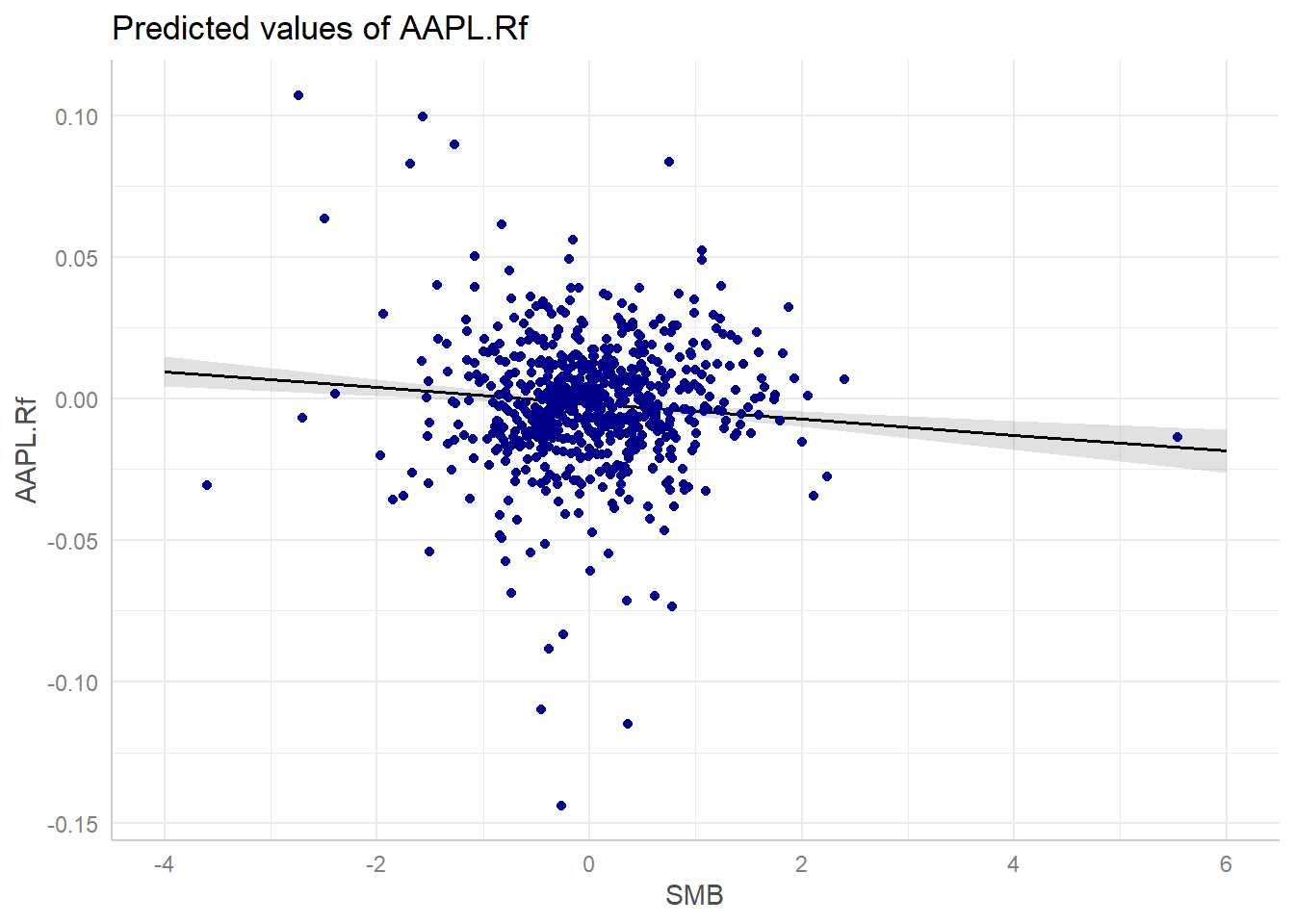Figure 8.3: Marginal Effect

# Topic 9 Panel Regression

• Panel data or longitudinal data is a data structure which contains individuals/variables (e.g., persons, firms, countries, cities etc) observed at several points in time (days, months, years, quarters etc).
• The dataset GDP_l.RData is an example of panel data where each country’s GDP is recorded over several years in time.
load("data/GDP_l.RData")
# data snapshot
GDP_l[c(1:5, 25:29, 241:245), ]
    Year   Country        GDP
1   1990 Australia 18247.3946
2   1991 Australia 18837.1893
3   1992 Australia 18599.0012
4   1993 Australia 17658.0794
5   1994 Australia 18080.6975
25  1990     China   314.4310
26  1991     China   329.7491
27  1992     China   362.8081
28  1993     China   373.8003
29  1994     China   469.2128
241 1990     World  4220.6460
242 1991     World  4357.3096
243 1992     World  4591.0928
244 1993     World  4604.2533
245 1994     World  4882.0794
• Some visualisation

• Line Chart

library(ggplot2)
p1 = ggplot(GDP_l, aes(Year, GDP, group = Country))

p1 + geom_path(aes(color = Country)) + theme_minimal() + theme(legend.position = "top")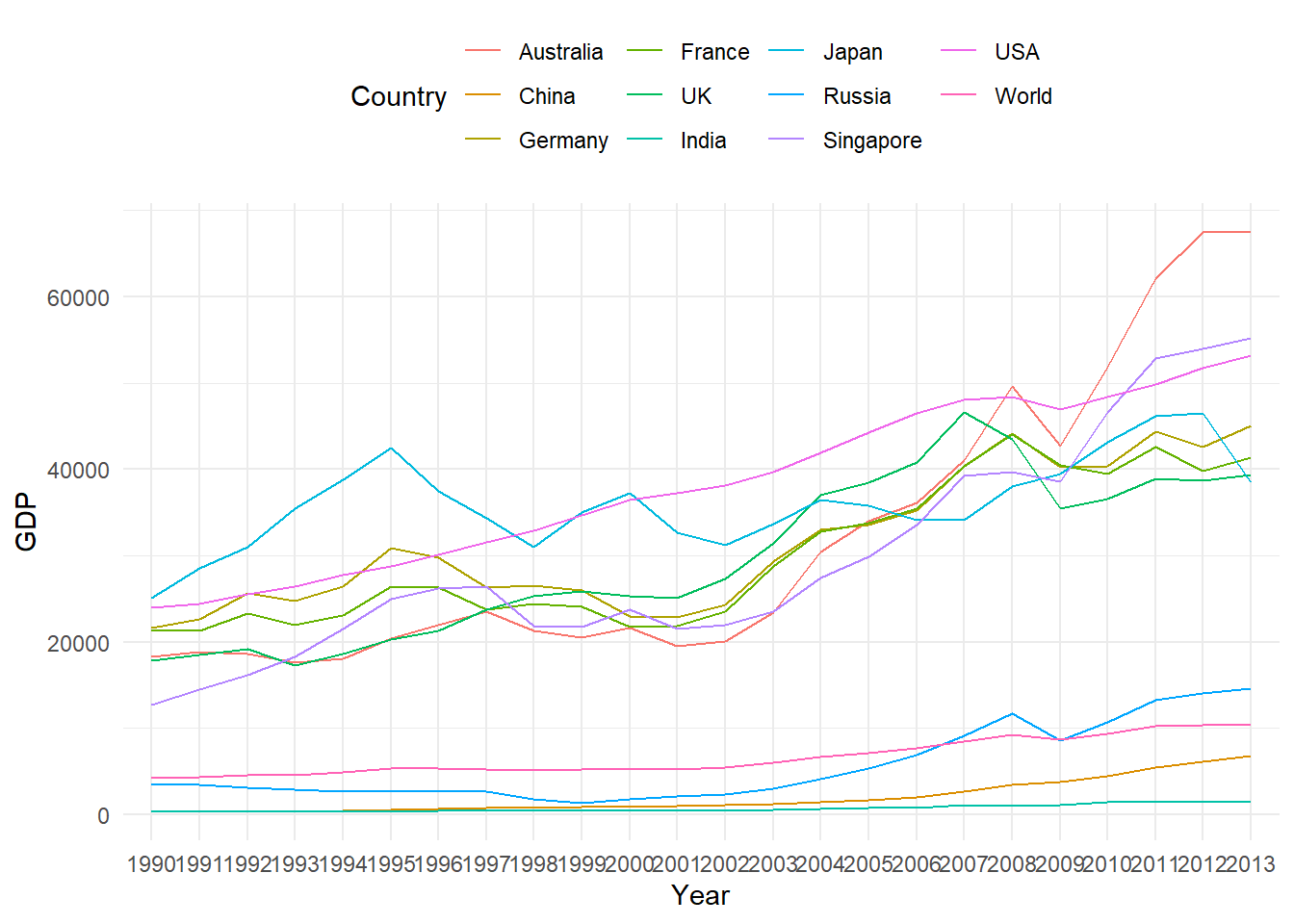Figure 9.1: Panel Data Line Chart

• Bar Chart
p1 + geom_col(aes(fill = Country)) + theme_minimal() + theme(legend.position = "top")Figure 9.2: Panel Data Bar Chart

• Bar Chart for each country
p1 + geom_col(aes(fill = Country)) + facet_grid(Country ~ .) + theme_minimal() +
theme(legend.position = "top")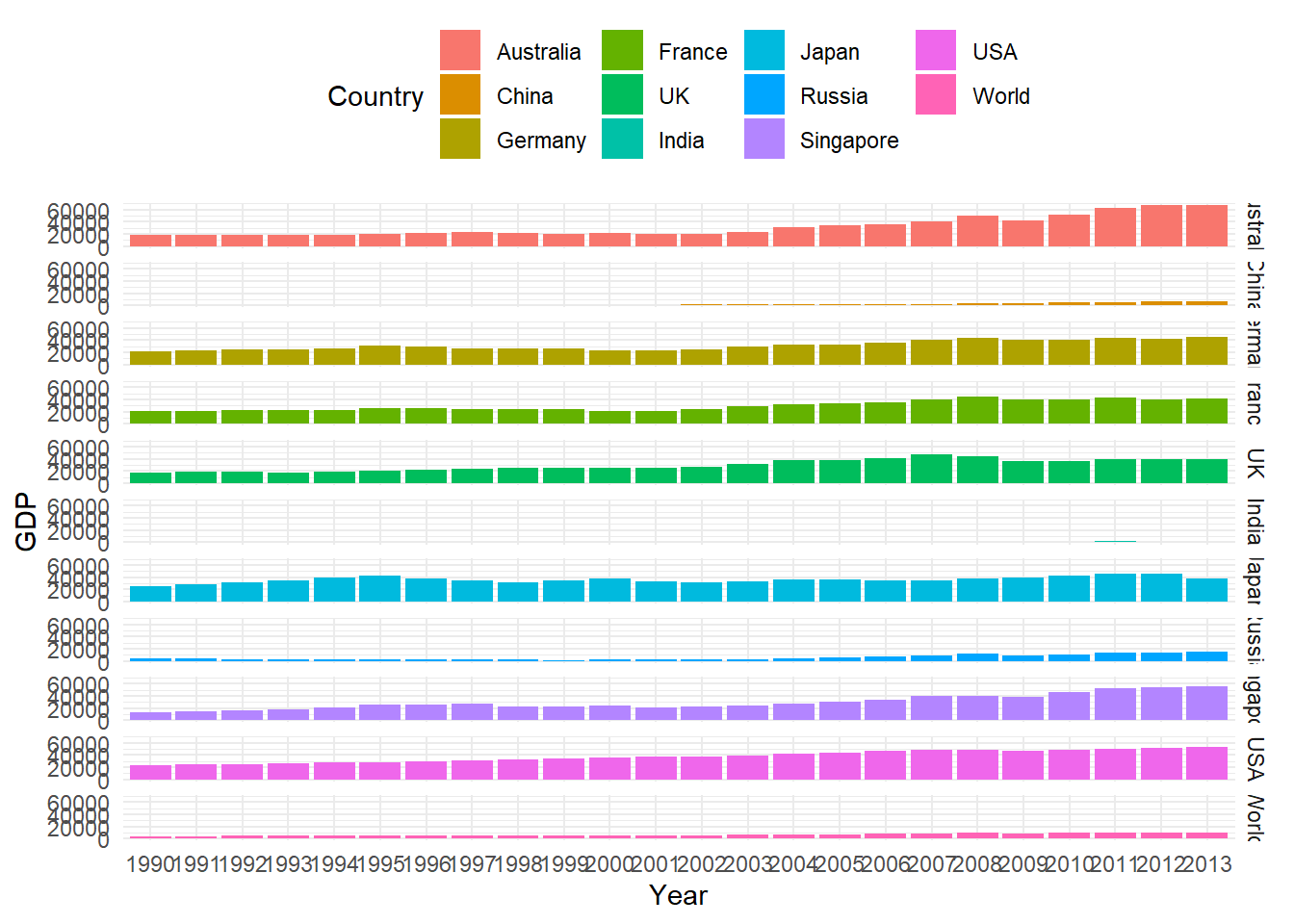Figure 9.3: Panel Data Bar Chart

• Box plot
p2 = ggplot(GDP_l, aes(Country, GDP))
p2 + geom_boxplot(aes(fill = Country)) + theme_minimal() + theme(legend.position = "top")Figure 9.4: Panel Data Box Plot

• The GDP data here has a balanced panel structure, where all the variables have values for all points in time.

• This chapter discussed the two basic panel regression models viz, Fixed Effect Model and Random Effect Model for balanced panel data.

• For an extensive discussion see econometrics textbooks including Baltagi (2005),Wooldridge (2010),Greene (2008) and Stock & Watson (2012).

• The package plm Croissant & Millo (2008) provides methods for calculating these models, which will be used for in illustrative code.

• We will use the very popular Grunfeld panel dataset Grunfeld (1958) available in the plm package for demostration which are based on similar examples in Croissant & Millo (2008) and Kleiber & Zeileis (2008).

## 9.1 Fixed and Random effects using the plm package

• The argument in is set to for fixed effects model and for a random effects model.

• The data can be converted to the required panel format using function, which transforms a regular data frame object into a panel data structure.

• is the main argument in the function which specifies the panel structure, i.e, columns with individual and time variables.

# Grunfeld Data representation as per pdata.frame function
library(plm)
head(Grunfeld)  #data snapshot
  firm year   inv  value capital
1    1 1935 317.6 3078.5     2.8
2    1 1936 391.8 4661.7    52.6
3    1 1937 410.6 5387.1   156.9
4    1 1938 257.7 2792.2   209.2
5    1 1939 330.8 4313.2   203.4
6    1 1940 461.2 4643.9   207.2
pdata1 = pdata.frame(Grunfeld, index = c("firm", "year"))
head(pdata1)
       firm year   inv  value capital
1-1935    1 1935 317.6 3078.5     2.8
1-1936    1 1936 391.8 4661.7    52.6
1-1937    1 1937 410.6 5387.1   156.9
1-1938    1 1938 257.7 2792.2   209.2
1-1939    1 1939 330.8 4313.2   203.4
1-1940    1 1940 461.2 4643.9   207.2

## 9.2 Fixed Effects Model

• We can use the plm package to replicate the following investment equation as considered by Grunfeld (Grunfeld, 1958).

$\begin{equation} I_{it}=\alpha+\beta_{1}F_{it}+\beta_{2}C_{it}+\varepsilon_{it} \tag{9.1} \end{equation}$

The model in equation-1 is a one-way panel regression model which attempts to quantify the dependence of real gross investment ($$I_{it}$$) on the real value of the company ($$F_{it}$$) and real value of its capital stock ($$C_{it}$$).

• Grunfeld (1958) studied 10 large manufacturing firms from the United States over 20 years (1935-954). A fixed effect estimation can be obtained with the following code
# using plm with 'within' estimator for fixed effects
fe1 = plm(inv ~ value + capital, data = pdata1, model = "within")
# the output can be summarised with summary
summary(fe1)
Oneway (individual) effect Within Model

Call:
plm(formula = inv ~ value + capital, data = pdata1, model = "within")

Balanced Panel: n = 10, T = 20, N = 200

Residuals:
Min.    1st Qu.     Median    3rd Qu.       Max.
-184.00857  -17.64316    0.56337   19.19222  250.70974

Coefficients:
Estimate Std. Error t-value  Pr(>|t|)
value   0.110124   0.011857  9.2879 < 2.2e-16 ***
capital 0.310065   0.017355 17.8666 < 2.2e-16 ***
---
Signif. codes:  0 '***' 0.001 '**' 0.01 '*' 0.05 '.' 0.1 ' ' 1

Total Sum of Squares:    2244400
Residual Sum of Squares: 523480
R-Squared:      0.76676
F-statistic: 309.014 on 2 and 188 DF, p-value: < 2.22e-16
• The summary output for the fitted model object gives details about the fitted object.

• The individual fixed effects can be obtained using the function , a summary method is also available as shown next

# individual fixed effects
fixef(fe1)
        1         2         3         4         5         6         7         8
-70.2967  101.9058 -235.5718  -27.8093 -114.6168  -23.1613  -66.5535  -57.5457
9        10
-87.2223   -6.5678 
# summary
summary(fixef(fe1))
    Estimate Std. Error t-value  Pr(>|t|)
1   -70.2967    49.7080 -1.4142   0.15896
2   101.9058    24.9383  4.0863 6.485e-05 ***
3  -235.5718    24.4316 -9.6421 < 2.2e-16 ***
4   -27.8093    14.0778 -1.9754   0.04969 *
5  -114.6168    14.1654 -8.0913 7.141e-14 ***
6   -23.1613    12.6687 -1.8282   0.06910 .
7   -66.5535    12.8430 -5.1821 5.629e-07 ***
8   -57.5457    13.9931 -4.1124 5.848e-05 ***
9   -87.2223    12.8919 -6.7657 1.635e-10 ***
10   -6.5678    11.8269 -0.5553   0.57933
---
Signif. codes:  0 '***' 0.001 '**' 0.01 '*' 0.05 '.' 0.1 ' ' 1

## 9.3 Random Effects Model

• A random effect model can be estimated by setting the argument to .

• There are five different methods available for estimation of the variance component (Baltagi (2005)) which can be selected using the argument.

• The following output is obtained using the default Swamy-Arora (Swamy & Arora (1972)) random method.

# random effect model
re1 = plm(inv ~ value + capital, data = pdata1, model = "random")
# summary
summary(re1)
Oneway (individual) effect Random Effect Model
(Swamy-Arora's transformation)

Call:
plm(formula = inv ~ value + capital, data = pdata1, model = "random")

Balanced Panel: n = 10, T = 20, N = 200

Effects:
var std.dev share
idiosyncratic 2784.46   52.77 0.282
individual    7089.80   84.20 0.718
theta: 0.8612

Residuals:
Min.   1st Qu.    Median   3rd Qu.      Max.
-177.6063  -19.7350    4.6851   19.5105  252.8743

Coefficients:
Estimate Std. Error z-value Pr(>|z|)
(Intercept) -57.834415  28.898935 -2.0013  0.04536 *
value         0.109781   0.010493 10.4627  < 2e-16 ***
capital       0.308113   0.017180 17.9339  < 2e-16 ***
---
Signif. codes:  0 '***' 0.001 '**' 0.01 '*' 0.05 '.' 0.1 ' ' 1

Total Sum of Squares:    2381400
Residual Sum of Squares: 548900
R-Squared:      0.7695
Chisq: 657.674 on 2 DF, p-value: < 2.22e-16

## 9.4 Testing

### 9.4.1 Panel or OLS

• It is important to test if the panel regression model is signficantly different from the OLS model. In other words, do we need a panel model or OLS model is good enough?

• The function in the plm package can be used to test a fitted fixed effect model against a fitted OLS model to check which regression is a better choice.

# Simple OLS (without the intercept) using pooling
ols1 = plm(inv ~ value + capital - 1, data = pdata1, model = "pooling")
# summary of results
summary(ols1)
Pooling Model

Call:
plm(formula = inv ~ value + capital - 1, data = pdata1, model = "pooling")

Balanced Panel: n = 10, T = 20, N = 200

Residuals:
Min. 1st Qu.  Median    Mean 3rd Qu.    Max.
-270.32  -51.32  -23.69  -21.04   -4.51  476.74

Coefficients:
Estimate Std. Error t-value  Pr(>|t|)
value   0.1076384  0.0058256 18.4769 < 2.2e-16 ***
capital 0.1832062  0.0242750  7.5471 1.587e-12 ***
---
Signif. codes:  0 '***' 0.001 '**' 0.01 '*' 0.05 '.' 0.1 ' ' 1

Total Sum of Squares:    9359900
Residual Sum of Squares: 1935600
R-Squared:      0.8113
F-statistic: 597.659 on 2 and 198 DF, p-value: < 2.22e-16
• The above OLS model can now be tested against the fixed effect model to check for the best fit.
# Testing for the better model, null: OLS is a better
pFtest(fe1, ols1)

F test for individual effects

data:  inv ~ value + capital
F = 50.714, df1 = 10, df2 = 188, p-value < 2.2e-16
alternative hypothesis: significant effects
• Similar to the fixed effect and OLS comparison one can also check if the random effects are needed using one of the available Langrange multiplier tests (Breusch & Pagan (1980) test here) test in function as illustrated below
# plmtest using the Breuch-Pagan method
plmtest(ols1, type = c("bp"))

Lagrange Multiplier Test - (Breusch-Pagan)

data:  inv ~ value + capital - 1
chisq = 727.84, df = 1, p-value < 2.2e-16
alternative hypothesis: significant effects
• A p-value<0.05 in the above test indicates that the Random Effect model is required.

### 9.4.2 Fixed Effect or Random Effect

• The Hausman test (Hausman (1978)) is the standard approach to test for model specification which can be computed using the function in the plm package.
# phtest using the fitted models in fe1 and re1
phtest(fe1, re1)

Hausman Test

data:  inv ~ value + capital
chisq = 2.3304, df = 2, p-value = 0.3119
alternative hypothesis: one model is inconsistent
• A p-value<0.05 suggests that the fixed effect model is appropriate so in this case the random effect model should be used.

### References

Baltagi, B. (2005). Econometric analysis of panel data (3rd ed.). John Wiley & Sons.
Breusch, T. S., & Pagan, A. R. (1980). The lagrange multiplier test and its applications to model specification in econometrics. The Review of Economic Studies, 239–253.
Croissant, Y., & Millo, G. (2008). Panel data econometrics in r: The plm package. Journal of Statistical Software, 27(2), 1–43.
Fama, E. F., & French, K. R. (1992). The cross-section of expected stock returns. The Journal of Finance, 47(2), 427–465.
Fama, E. F., & French, K. R. (1993). Common risk factors in the returns on stocks and bonds. Journal of Financial Economics, 33(1), 3–56.
Greene, W. H. (2008). Econometric analysis. Granite Hill Publishers.
Grunfeld, Y. (1958). The determinants of corporate investment: A study of a number of large corporations in the united states (PhD thesis). Department of Photoduplication, University of Chicago Library.
Hausman, J. (1978). Specification tests in econometrics. Econometrica.
Kleiber, C., & Zeileis, A. (2008). Applied econometrics with r. Springer Science & Business Media.
Stock, J. H., & Watson, M. W. (2012). Introduction to econometrics: Global edition. Pearson Education.
Swamy, P., & Arora, S. S. (1972). The exact finite sample properties of the estimators of coefficients in the error components regression models. Econometrica: Journal of the Econometric Society, 261–275.
Wooldridge, J. M. (2010). Econometric analysis of cross section and panel data. MIT press.